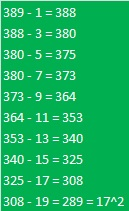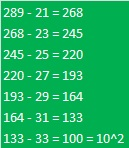# 389 How to Know if a Prime Number is the Hypotenuse in a Primitive Pythagorean Triple

• 389 is a prime number.
• Prime factorization: 389 is prime.
• The exponent of prime number 389 is 1. Adding 1 to that exponent we get (1 + 1) = 2. Therefore 389 has exactly 2 factors.
• Factors of 389: 1, 389
• Factor pairs: 389 = 1 x 389
• 389 has no square factors that allow its square root to be simplified. √389 ≈ 19.723How do we know that 389 is a prime number? If 389 were not a prime number, then it would be divisible by at least one prime number less than or equal to √389 ≈ 19.723. Since 389 cannot be divided evenly by 2, 3, 5, 7, 11, 13, 17, or 19, we know that 389 is a prime number.

If a Pythagorean triple is a primitive, then its hypotenuse is always an odd number. Sometimes it is also a prime number. How can we know if a particular prime number, such as 389, is ever the hypotenuse of a Pythagorean triple?

Divide the last two digits of the prime number by 4 and look at the remainder:

• If the remainder is 3, then that prime number will not ever be the hypotenuse of any Pythagorean triple.
• If the remainder is 1, then it is the hypotenuse of a primitive Pythagorean triple! Find the proof here.

The last two digits of 389 is 89, and 89 ÷ 4 = 22 R1, so 389 and every other prime number ending in 89 is the hypotenuse of a primitive Pythagorean triple. What are the other two sides of this particular triangle? First we will figure out what two square numbers equal 389, then we will use those two numbers to figure out what two square numbers equal 389 squared:

Have you ever noticed that 1 is the first square number, 1 + 3 or 4 is also a square number, and 1 + 3 + 5 or 9 is yet another square number? This principle can be summarized by writing 1 + 3 + 5 + . . . + (2n – 1) = n squared.

I’ll use this fact to determine when 389 is the hypotenuse of a primitive Pythagorean triple. I’ll subtract 1 from 389, then 3 from that result, and continue to subtract the next odd number until I get a perfect square. I should find the perfect square before I reach 194 (about half of 389).

So let’s begin:At this point I know that 17² + another square equals 389. There are a few methods I can use to find the other perfect square.

• I can find the other square by subtracting: 389 – 17²
• I can take the last odd number used, 19, add one, then divide by two and then square that number.
• Or I can continue subtracting odd numbers as before:All of these methods give us 100 or 10² as the second perfect square. Notice that (33 + 1)/2 = 17 as well.

Now we have enough information to determine the other two numbers that make up the Pythagorean triple with 389 as the hypotenuse.

• All of the numbers, a – b – c, in the triple can be found using the formulas Euclid provided more than 2000 years ago. Let 10 = n; and 17 = m.
• a = m² – n² = 17² – 10² = 189
• b = 2mn = 2(10)(17) = 340
• c = m² + n² = 17² + 10² = 389
• thus 189 – 340 – 389 make a primitive Pythagorean triple. We know it is a primitive because 389 is prime and has no other factors besides one and itself.

## 2 thoughts on “389 How to Know if a Prime Number is the Hypotenuse in a Primitive Pythagorean Triple”

1.Steve Morris

Clever!

•ivasallay

Thank you!

This site uses Akismet to reduce spam. Learn how your comment data is processed.